# OliNo

Renewable Energy

## Luxerna TL ECO 80 865 smd 120cm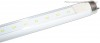presents a 120 cm long led tube with 60 smd-leds mounted.

This article shows the measurement results. Many parameters are also found in the Eulumdat file.

See this overview for a comparison with other light bulbs.

### Summary measurement data

parameter meas. result remark
Color temperature 6777 K cold white
Luminous intensity Iv 438 Cd Measured straight underneath the lamp
Beam angle 124 deg 123º for the C0-C180 plane (crosses the length direction of the tube), and 124º for C90-C270 plane (along the length direction of tube).
Power P 19.1 W
Power Factor 0.93 For every 1 kWh net power consumed, there has been 0.4 kVAhr for reactive power.
Luminous flux 1382 Lm
Luminous efficacy 72 Lm/W
CRI_Ra 77 Color Rendering Index.
Coordinates chromaticity diagram x=0.3102 and y=0.3143
Fitting FL-tube This tube is used in a normal luminaire for fluorescent tube light, where the starter is exchanged for a new type that shorts its pins.
PAR-value 4.2 μMol/s/m2 The number of photons seen by an average plant when it is lit by the light of this light bulb. Value valid at 1 m distance from light bulb.
PAR-photon efficacy 0.7 μMol/s/We The toal emitted number of photons by this light, divided by its consumption in W. It indicates a kind of efficacy in generating photons.
S/P ratio 2.1 This factor indicates the amount of times more efficient the light of this light bulb is perceived under scotopic circumstances (ow environmental light level).
D x L external dimensions 26 x 1197 mm External dimensions of the tube (D = diameter). Excluding the pins.
L x W luminous area 1115 x 13 mm Dimensions of the luminous area (used in Eulumdat file). This is equal to the surface of the plate on which the leds are mounted.
General remarks The ambient temperature during the whole set of measurements was 23-25 deg C. The maximum temperature of the tube light gets about 20 degrees hotter than ambient.
Warm up effect: during the warm up time the illuminance decreases with ≈6 % and the consumed power with ≈6 %.

Voltage dependency: the power consumption and illuminance do not significantly depend on the voltage when it is varied from 200 – 250 V.

Measurement report (PDF)Eulumdat fileRight click on icon and save the file.

### Overview table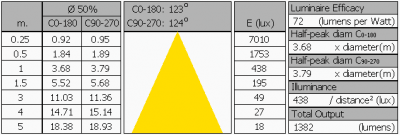The overview table is explained on the OliNo website. Please note that this overview table makes use of calculations, use this data with care as explained on the OliNo site. E (lux) values are not accurate, when within 5 x 1115 mm = 5575 mm. Measured lux values willl be less than the computed values in this overview as the measurements are then within the near field of the tube.

### Eulumdat light diagram

This light diagram below comes from the program Qlumedit, that extracts these diagrams from an Eulumdat file. It is explained on the OliNo site.The light diagram giving the radiation pattern.

It indicates the luminous intensity around the light bulb. In the length direction (C90-C270) the beam angle is the same as in the direction crossing the length direction (C0-C180).

### Illuminance Ev at 1 m distance, or luminous intensity Iv

Herewith the plot of the averaged luminous intensity Iv as a function of the inclination angle with the light bulb.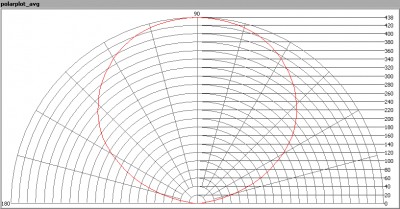The radiation pattern of the light bulb.

This radiation pattern is the average of the light output of the light diagram given earlier. Also, in this graph the luminous intensity is given in Cd.

These averaged values are used (later) to compute the lumen output.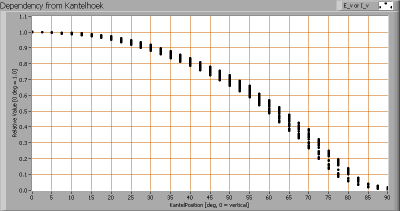Intensity data of every measured turn angle at each inclination angle.

This plot shows per inclination angle the intensity measurement results for each turn angle at that inclination angle. There normally are differences in illuminance values for different turn angles. However for further calculations the averaged values will be used.

When using the average values per inclination angle, the beam angle can be computed, being 123-124º depending on the reference plane.

### Luminous flux

With the averaged illuminance data at 1 m distance, taken from the graph showing the averaged radiation pattern, it is possible to compute the luminous flux.

The result of this computation for this light spot is a luminous flux of 1382 Lm.

### Luminous efficacy

The luminous flux being 1382 Lm, and the power of the light bulb being 19.1 W, yields a luminous efficacy of 72 Lm/W.

A power factor of 0.93 means that for every 1 kWh net power consumed, a reactive component of 0.4 kVAr was needed.

 Light bulb voltage (used on power supply!) 230 VAC Light bulb current 90 mA Power P 19.1 W Apparent power S 20.6 VA Power factor 0.93

Of this light bulb the voltage across ad the resulting current through it are measured and graphed. See the OliNo site how this is obtained.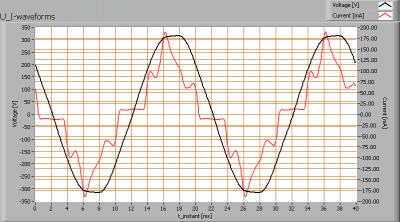Voltage across and current through the lightbulb

The current does not have a sine form, but the power supply tries to approach it. The current phase is a bit leading the voltage’s. The powerfactor is very good with a value near 0.95.

Also the power spectrum of the current is determined.Squared amplitudes of higher harmonics related to the first harmonic (50 Hz).

Due to the steep edges and peaks in the current there are a number of higher harmonics.

The Total Harmonic Distortion of the current is 36 %.

### Temperature measurements lamp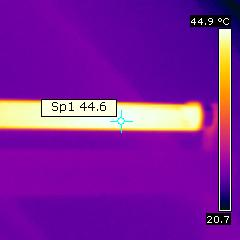Temperature image after warm up period, warmest spot on the tube

Tape is put on the hotspot and then zoomed in to measure its temperature.

 status lamp > 2 hours on ambient temperature 23.5 deg C reflected background temperature 23.5 deg C camera Flir B-CAM Western S emissivity 0.95(1) measurement distance 0.35 m IFOVgeometric 1.3 mm NETD (thermal sensitivity) 100 mK

(1) The emissivity of the masking tape is used which is about 0.95. The masking tape is so thin that it quickly takes the same temperature as the light bulb’s heat sink.

The tube on its hotttest place gets no warmer than warm-to-the-touch.

### Color temperature and Spectral power distribution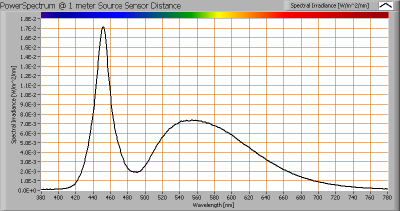The spectral power distribution of this light bulb, energies on y-axis valid at 1 m distance.

The measured color temperature is about 6775 K which is cold white.

This color temperature is measured straight underneath the light bulb. Below a graph showing the color temperature for different inclination angles.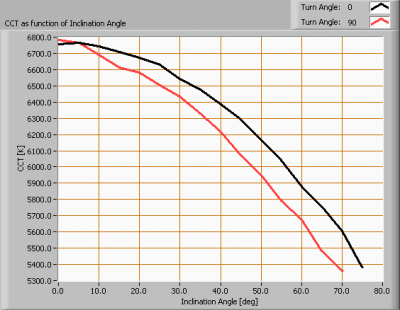Color temperature as a function of inclination angle.

The measurement of CCT is measured for inclination angles up to 70º since then the illuminance value was decreased to very low values (< 5 lux).

The beam angle is maximally 124º, meaning a 62º inclination angle. In this area the majority of the light is present. The variation in correlated color temperature in this area is about 18 %.

### PAR value and PAR spectrum

To make a statement how well the light of this light bulb is for growing plants, the PAR-area needs to be determined. See the OliNo website how this all is determined and the explanation of the graph.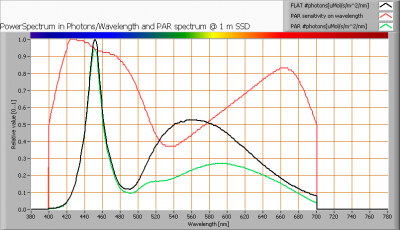The photon spectrum, then the sensitivity curve and as result the final PAR spectrum of the light of this light bulb

parameter value unit
PAR-number 4.2 μMol/s/m²
PAR-photon current 13.2 μMol/s
PAR-photon efficacy 0.7 μMol/s/W

The PAR efficiency is 67 % (valid for the PAR wave length range of 400 – 700 nm). So maximally 67 % of the total of photons in the light is effectively used by the average plant (since the plant might not take 100 % of the photons at the frequency where its relative sensitivity is 100 %).

### S/P ratio

The S/P ratio and measurement is explained on the OliNo website. Here the results are given.The power spectrum, sensitivity curves and resulting scotopic and photopic spectra (spectra energy content defined at 1 m distance).

The S/P ratio is 2.1

More info on S/P ratio can be found on the OliNo website.

### Chromaticity diagram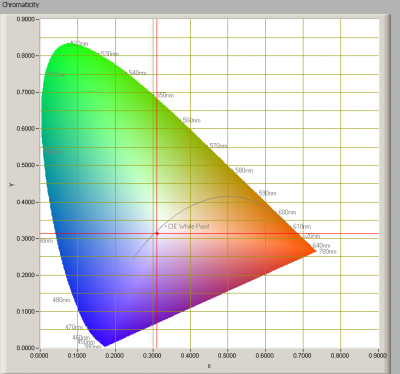The chromaticity space and the position of the lamp’s color coordinates in it.

The light coming from this lamp is close to the Planckian Locus (the black path in the graph).

Its coordinates are x=0.3102 and y=0.3143.

### Color Rendering Index (CRI) or also Ra

Herewith the image showing the CRI as well as how well different colors are represented (rendered). The higher the number, the better the resemblance with the color when a black body radiator would have been used (the sun, or an incandescent lamp). Practical information and also some critics about the CRI can be found on the OliNo website.

Each color has an index Rx, and the first 8 indexes (R1 .. R8) are averaged to compute the Ra which is equivalent to the CRI.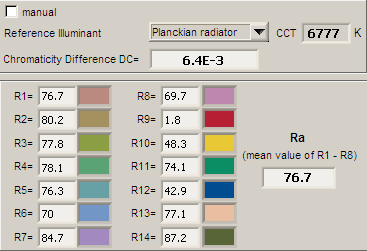CRI of the light of this lightbulb.

The value of 77 is lower than 80 which is considered a minimum value for indoor usage.

Note: the chromaticity difference is 0.0064 indicates the distance to the Planckian Locus. Its value is higher than 0.0054, which means that the calculated CRI result is not meaningful.

### Voltage dependency

The dependency of a number of lamp parameters on the lamp voltage is determined. For this, the lamp voltage has been varied and its effect on the following light bulb parameters measured: illuminance E_v [lx], the lamppower P [W], the (Correlated) Color Temperature [K] and the luminous efficacy [Lm/W].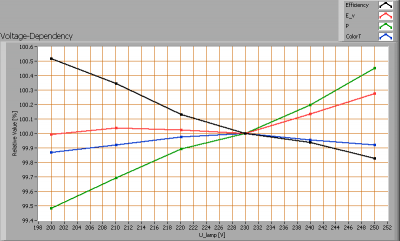Lamp voltage dependencies of certain light bulb parameters, where the value at 230 V is taken as 100 %.

The illuminance and consumed power do not vary significantly when the voltage is varied.

When the voltage at 230 V varies with + and – 5 V, then the illuminance varies < 0.1 %, so when abrupt voltage changes occur this effect is not visible in the illuminance output.

### Warm up effects

After switch on of a cold lamp, the effect of heating up of the lamp is measured on illuminance E_v [lx], color temperature CT or correlated color temperature CCT [K], the lamppower P [W] and the luminous efficacy [lm/W].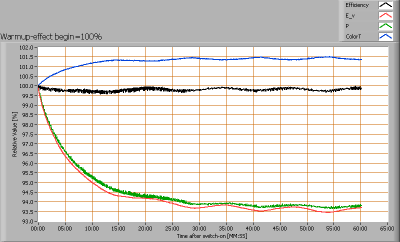Effect of warming up on different light bulb parameters. At top the 100 % level is put at begin, and at bottom at the end.

The warm up time is about 30 minutes. During that time the illuminance decreases with ≈ 6 % and the consumed power with ≈ 6 %.